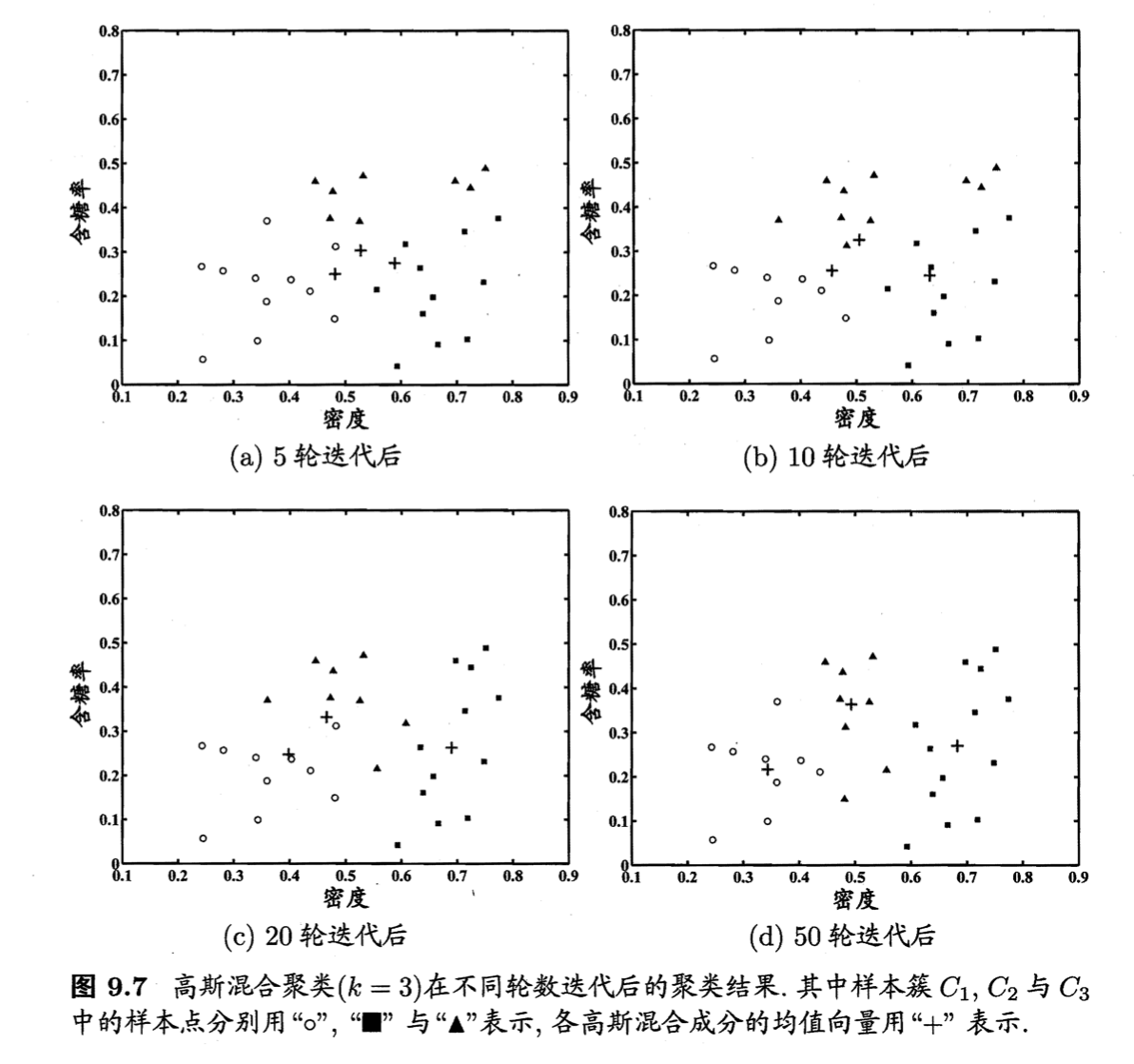# 【机器学习基础】第三十五课：聚类之原型聚类

## 原型聚类，k均值算法，学习向量量化（LVQ），Voronoi剖分，高斯混合聚类

Posted by x-jeff on March 21, 2022

【机器学习基础】系列博客为参考周志华老师的《机器学习》一书，自己所做的读书笔记。

# 1.原型聚类

“原型”是指样本空间中具有代表性的点。

# 2.k均值算法

$E=\sum^k_{i=1} \sum_{\mathbf{x} \in C_i} \parallel \mathbf{x} - \mathbf{\mu}_i \parallel_2^2 \tag{1}$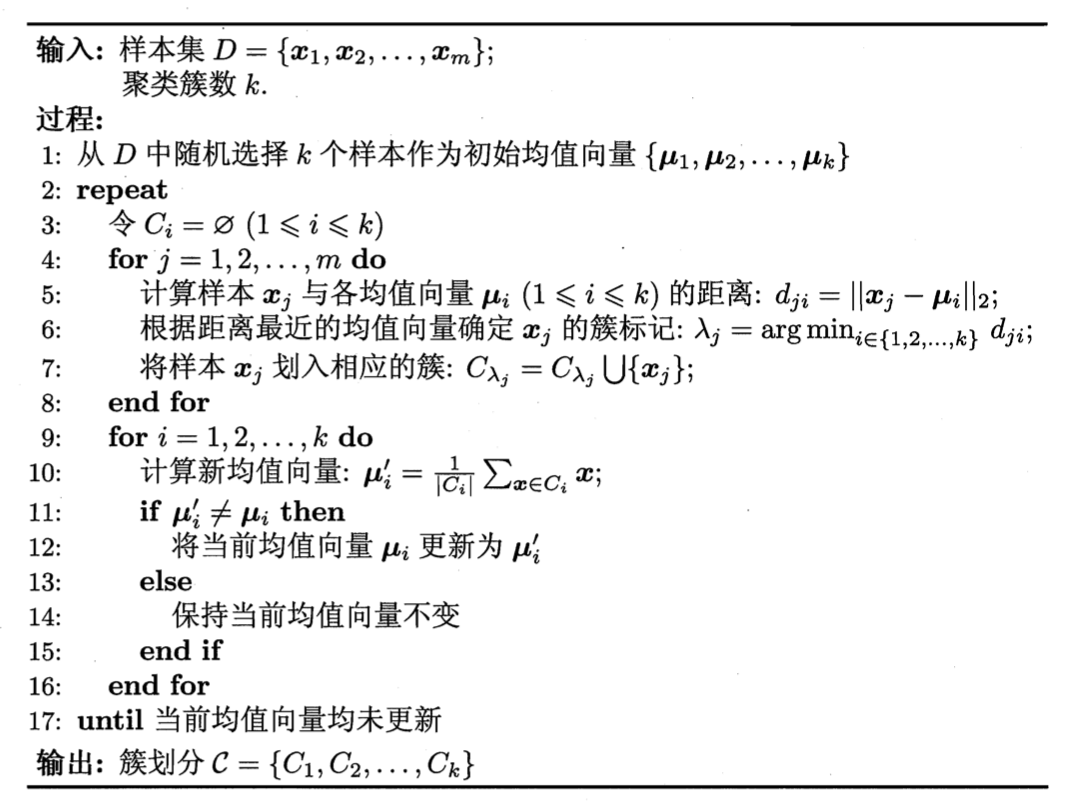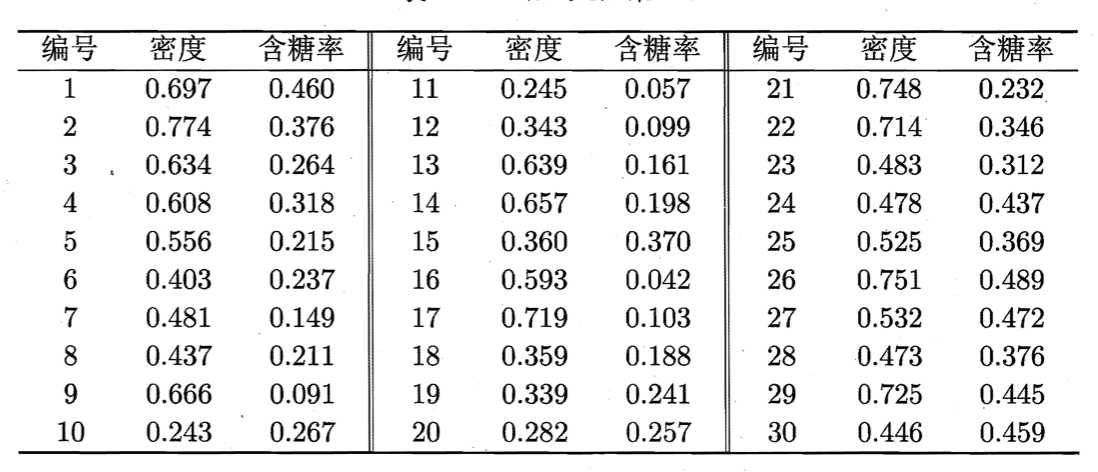$\mathbf{\mu}_1=(0.403;0.237 ), \mathbf{\mu}_2=(0.343;0.099),\mathbf{\mu}_3=(0.532;0.472)$

$C_1=\{\mathbf{x}_5,\mathbf{x}_6,\mathbf{x}_7,\mathbf{x}_8,\mathbf{x}_9,\mathbf{x}_{10},\mathbf{x}_{13},\mathbf{x}_{14},\mathbf{x}_{15},\mathbf{x}_{17},\mathbf{x}_{18},\mathbf{x}_{19},\mathbf{x}_{20},\mathbf{x}_{23} \}$ $C_2=\{\mathbf{x}_{11},\mathbf{x}_{12},\mathbf{x}_{16} \}$ $C_3 = \{\mathbf{x}_1,\mathbf{x}_2,\mathbf{x}_3,\mathbf{x}_4,\mathbf{x}_{21},\mathbf{x}_{22},\mathbf{x}_{24},\mathbf{x}_{25},\mathbf{x}_{26},\mathbf{x}_{27},\mathbf{x}_{28},\mathbf{x}_{29},\mathbf{x}_{30} \}$

$\mathbf{\mu}'_1=(0.473;0.214),\mathbf{\mu}'_2=(0.394;0.066),\mathbf{\mu}'_3=(0.623;0.388)$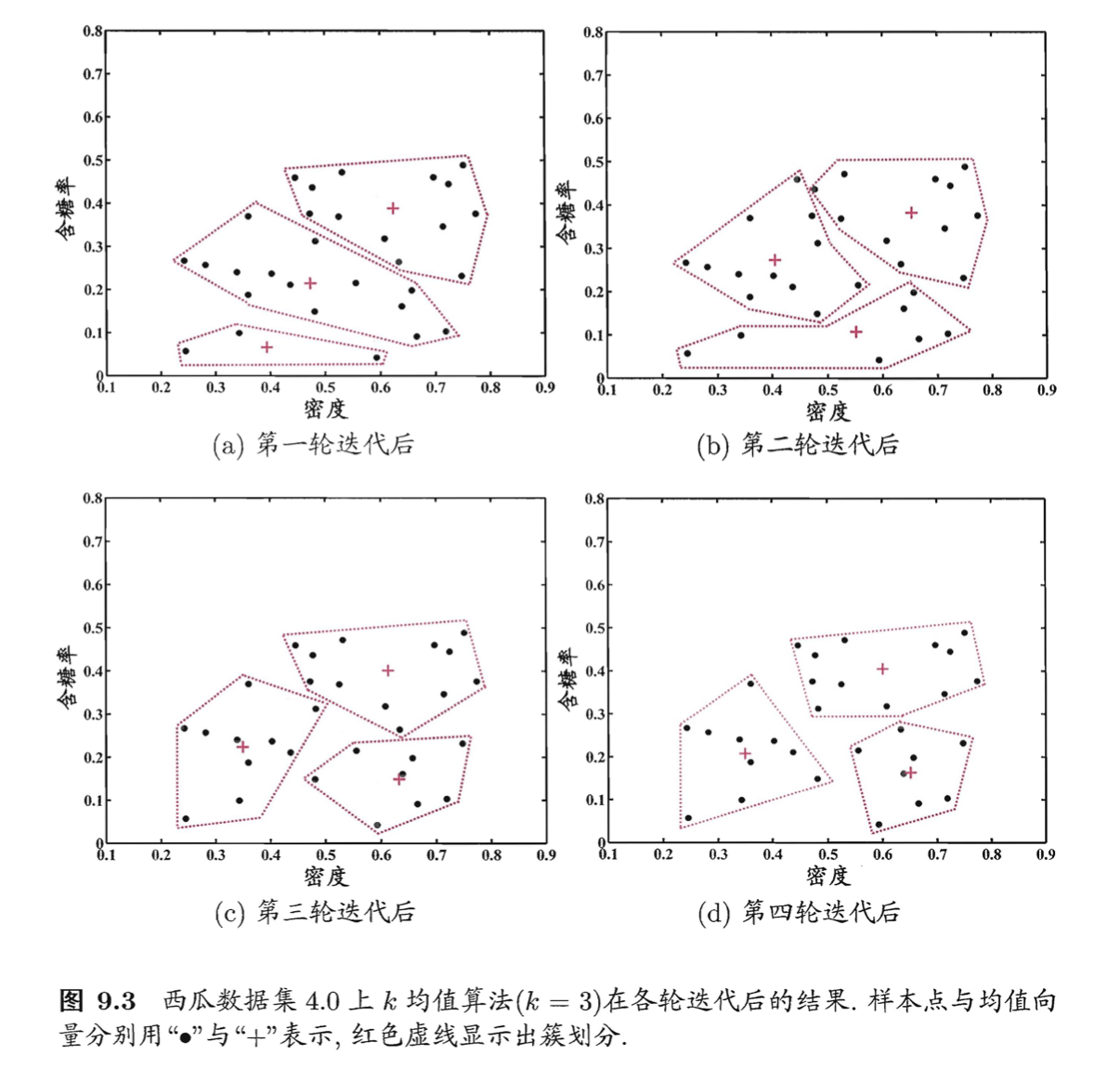# 3.学习向量量化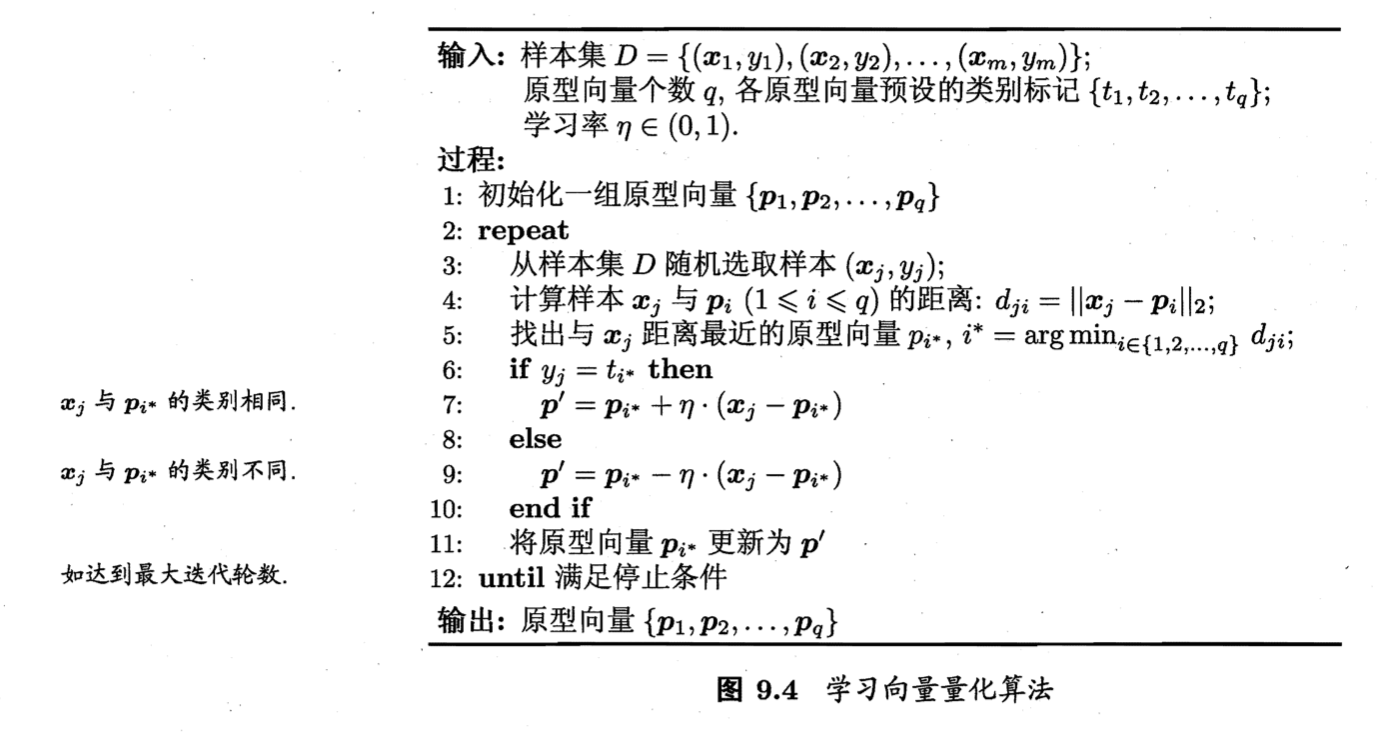LVQ算法描述如上图所示，算法第1行先对原型向量进行初始化，例如对第$q$个簇可从类别标记为$t_q$的样本中随机选取一个作为原型向量。算法第2～12行对原型向量进行迭代优化。在每一轮迭代中，算法随机选取一个有标记训练样本，找出与其距离最近的原型向量，并根据两者的类别标记是否一致来对原型向量进行相应的更新。在第12行中，若算法的停止条件已满足（例如已达到最大迭代轮数，或原型向量更新很小甚至不再更新），则将当前原型向量作为最终结果返回。

$\mathbf{p}' = \mathbf{p}_{i^*}+\eta \cdot (\mathbf{x}_j - \mathbf{p}_{i^*}) \tag{2}$

$\mathbf{p}’$与$\mathbf{x}_j$之间的距离为：

\begin{align} \parallel \mathbf{p}' - \mathbf{x}_j \parallel_2 &= \parallel \mathbf{p}_{i^*}+\eta \cdot (\mathbf{x}_j - \mathbf{p}_{i^*}) - \mathbf{x}_j \parallel_2 \\&= (1-\eta) \cdot \parallel \mathbf{p}_{i^*} - \mathbf{x}_j \parallel _2 \end{align} \tag{3}

$R_i = \{ \mathbf{x} \in \chi \mid \parallel \mathbf{x}-\mathbf{p}_i \parallel_2 \leqslant \parallel \mathbf{x} - \mathbf{p}_{i'} \parallel_2, i' \neq i \} \tag{4}$

\begin{align} \mathbf{p}' &= \mathbf{p}_5 + \eta \cdot (\mathbf{x}_1 - \mathbf{p}_5) \\&= (0.725;0.445) + 0.1 \cdot ((0.697;0.460)-(0.725;0.445)) \\&= (0.722;0.442) \end{align}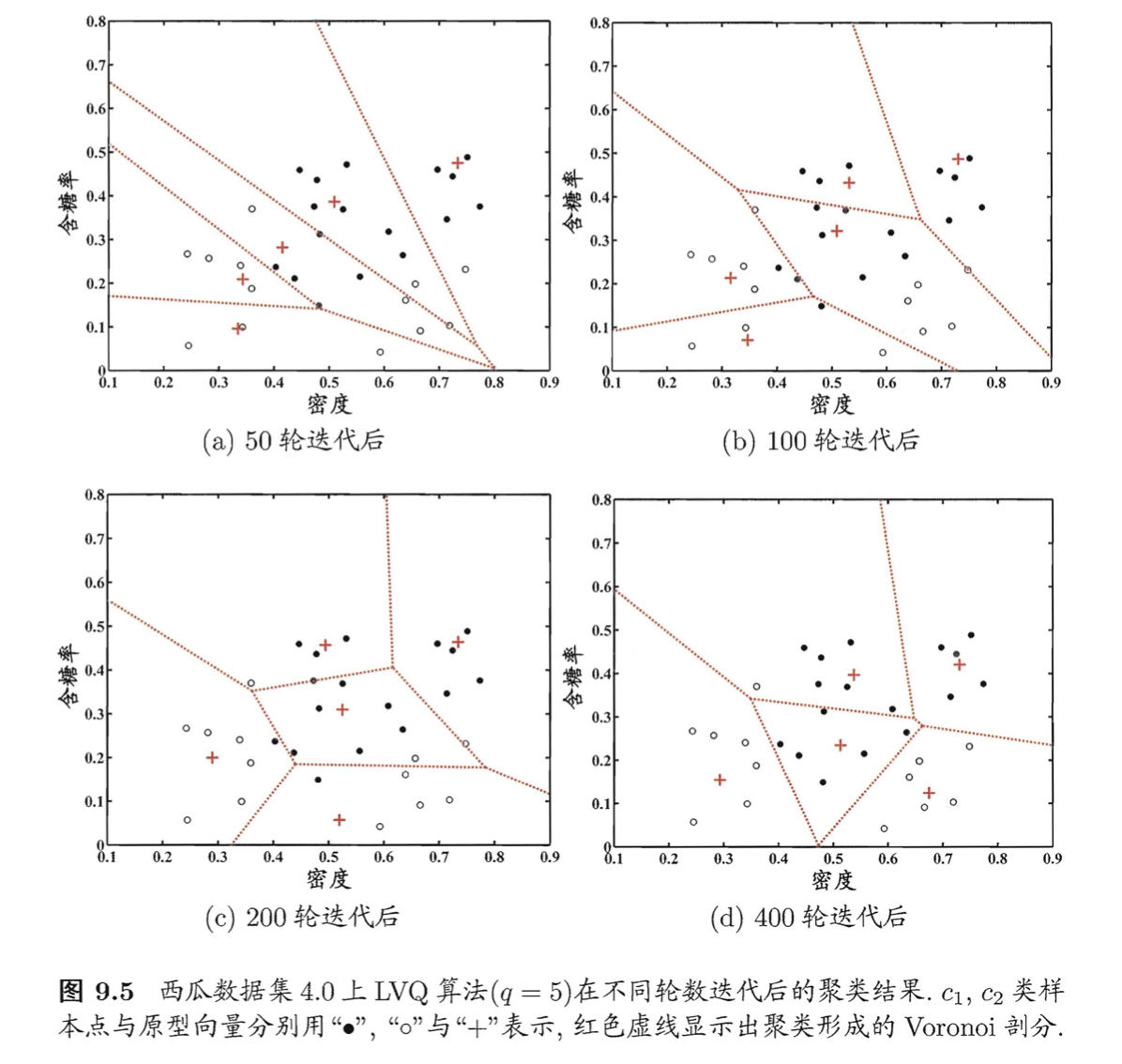# 4.高斯混合聚类

$p(\mathbf{x})=\frac{1}{(2\pi)^{\frac{n}{2}} \lvert \Sigma \rvert ^{\frac{1}{2}}} e^{-\frac{1}{2} (\mathbf{x} - \mathbf{\mu})^T \Sigma^{-1} (\mathbf{x} - \mathbf{\mu})} \tag{5}$

$\Sigma$：对称正定矩阵
$\lvert \Sigma \rvert$：$\Sigma$的行列式
$\Sigma^{-1}$：$\Sigma$的逆矩阵

$p_{\mathcal{M}}(\mathbf{x})=\sum^k_{i=1}\alpha_i \cdot p(\mathbf{x} \mid \mathbf{\mu}_i,\Sigma_i) \tag{6}$

$p_{\mathcal{M}}(\cdot)$也是概率密度函数，$\int p_{\mathcal{M}}(\mathbf{x})d\mathbf{x}=1$。

\begin{align} p_{\mathcal{M}}(z_j =i \mid \mathbf{x}_j) &= \frac{P(z_j=i) \cdot p_{\mathcal{M}}(\mathbf{x}_j \mid z_j = i)}{p_{\mathcal{M}}(\mathbf{x}_j)} \\&= \frac{\alpha_i \cdot p(\mathbf{x}_j \mid \mathbf{\mu}_i,\Sigma_i) }{\sum^k_{l=1} \alpha_l \cdot p( \mathbf{x}_j \mid \mathbf{\mu}_l,\Sigma_l ) } \end{align} \tag{7}

$\lambda_j = \mathop{\arg \max} \limits_{i \in \{1,2,...,k \}} \gamma_{ji} \tag{8}$

\begin{align} LL(D) &= \ln \left( \prod^m_{j=1} p_{\mathcal{M}}(\mathbf{x}_j) \right) \\&= \sum^m_{j=1} \ln \left( \sum^k_{i=1} \alpha_i \cdot p(\mathbf{x}_j \mid \mathbf{\mu}_i,\Sigma_i ) \right) \end{align} \tag{9}

$\mathbf{\mu}_i = \frac{\sum^m_{j=1} \gamma_{ji} \mathbf{x}_j}{\sum^m_{j=1} \gamma_{ji}} \tag{10}$

$\Sigma_i = \frac{ \sum^m_{j=1} \gamma_{ji} (\mathbf{x}_j - \mathbf{\mu} _i) (\mathbf{x}_j - \mathbf{\mu} _i)^T }{\sum^m_{j=1} \gamma_{ji}} \tag{11}$

$LL(D)+\lambda\left( \sum^k_{i=1} \alpha_i - 1 \right) \tag{12}$

$\alpha_i = \frac{1}{m} \sum^m_{j=1} \gamma_{ji} \tag{13}$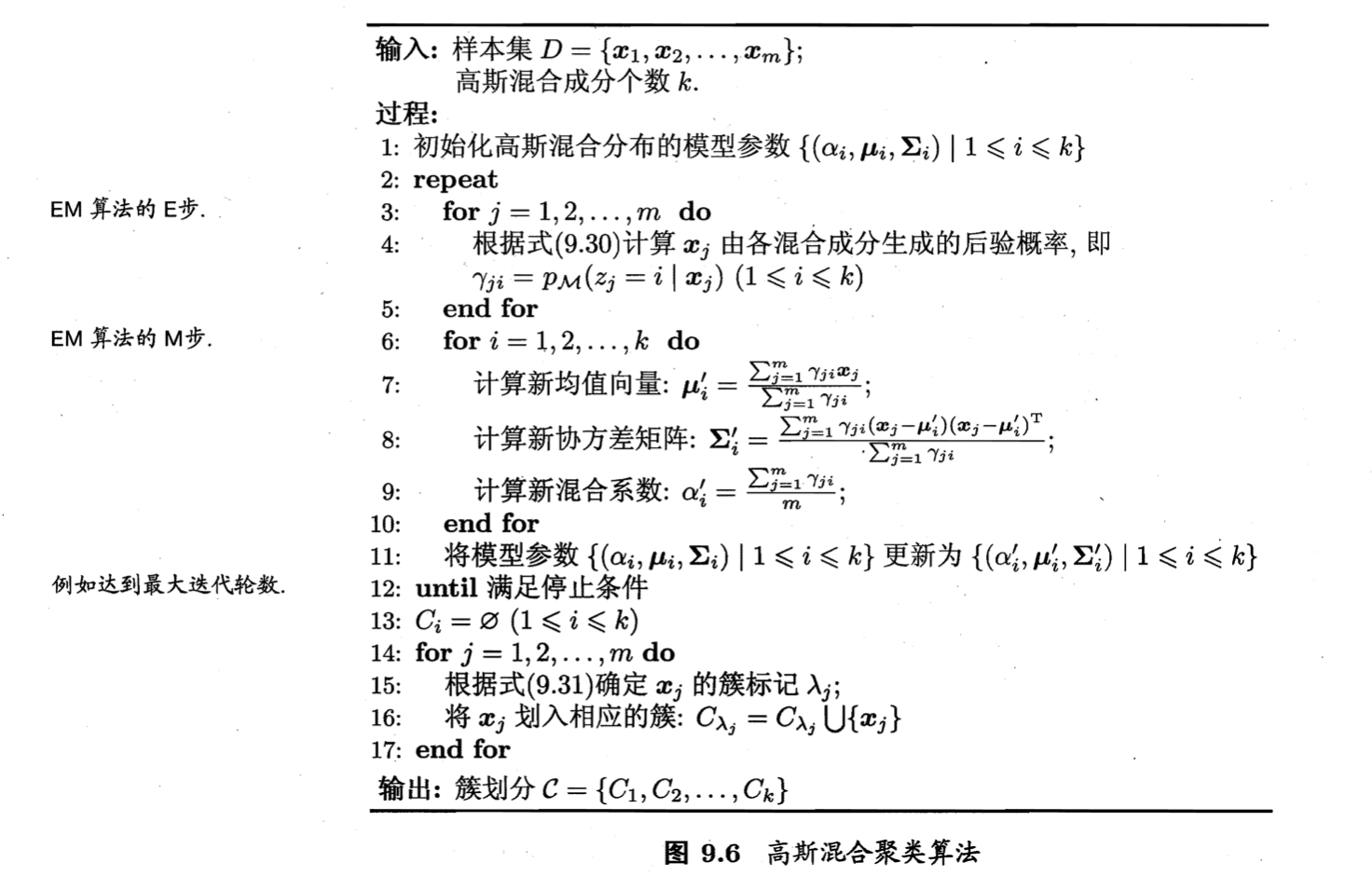EM算法停止条件可以是已达到最大迭代轮数，或似然函数$LL(D)$增长很少甚至不再增长。

$\alpha_1 = \alpha_2 = \alpha_3 = \frac{1}{3}$ $\mathbf{\mu}_1 = \mathbf{x}_6, \mathbf{\mu}_2 = \mathbf{x}_{22}, \mathbf{\mu}_3 = \mathbf{x}_{27}$ $\Sigma_1=\Sigma_2=\Sigma_3=\begin{pmatrix} 0.1 & 0.0 \\ 0.0 & 0.1 \\ \end{pmatrix}$

$\alpha_1'=0.361,\alpha_2'=0.323,\alpha_3'=0.316$ $\mathbf{\mu}_1'=(0.491;0.251), \mathbf{\mu}_2'=(0.571;0.281),\mathbf{\mu}_3'=(0.534;0.295)$ $\Sigma_1'=\begin{pmatrix} 0.025 & 0.004 \\ 0.004 & 0.016 \\ \end{pmatrix}, \Sigma_2'=\begin{pmatrix} 0.023 & 0.004 \\ 0.004 & 0.017 \\ \end{pmatrix}, \Sigma_3' = \begin{pmatrix} 0.024 & 0.005 \\ 0.005 & 0.016 \\ \end{pmatrix}$